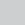Email Support | Mon - Fri: 9am - 5pm Sun: 12pm - 4pm CT | Call Support: 312-775-7009

# Square Challenge Coin Holder

2 likes
0 opens
0 copies

## General Information

Basic square challenge coin holder made from cedar wood.

Attribution 3.0 Unported (CC BY 3.0)
Like this project
File Description Unit Price### Details for two coin holders cut at the same time.docx

Details of build parameters

\$0

\$0
from Inventables

1

## 1st Layer

60 minutes

Within Easel:
1st Layer (file 11X11 Square Coin Holder)(https://www.inventables.com/projects/11×11-square-coin-holder) Details below for left most square coin holder. Files show two coin holders- remove the second one as needed.
-Set material dimensions (I used X=23.0625 in, Y=11.4735 in, Z=0.79 in)
-Cut settings 40 in/min, depth 0.0825”/pass (cedar)
-(outer edge(OE)) Click rectangle tab, set details in opened tab:
X=0.25
Y= 0
Width (W) =11”
Height (H) = 11”
Cut depth 0.79
-(outer box(OB))Click rectangle tab, set:
X=0.75
Y=0.50
W= 10
H = 10
Cut depth= 0.385
- (inner box(IB)) Click rectangle tab, set:
X=1.125
Y=0.8750
W=9.25
H= 9.25
Cut depth = 0
-Select the two (outer box, inner box) boxes using shift key and mouse, click ‘edit’ and ‘combine’ from pull down menu. (creates the 0.375” wide channel, 0.385” deep)
-Verify cut depth 0.385” on new object (combined box)
- Click “outer edge”, click ‘edit’ and ‘send to back’
- Click “combined box”, click ‘edit’ and ‘send to front’

2

## 2nd Layer

40 minutes

Layer 2: (file 9.25×9.25 Square Coin Holder) (https://www.inventables.com/projects/925×925-square-coin-holder)
This layer follows the same process as layer 1 with these changes:
(OE)
X= 0.25
Y=0.25
W= 9.25
H=9.25
Z=0.79
(OB)
X=0.75
Y=0.75
W=8.25
H=8.25
(IB)
X=1.125
Y=1.25
W=7.50
H=7.50

3

## Layer 3

30 minutes

Layer 3 (file 7.5×7.5 Square Coin Holder) (https://www.inventables.com/projects/75×75-square-coin-holder)
(OE)
X= 0.25
Y=0.50
W= 7.5
H=7.5
Z=0.79
(OB)
X=0.75
Y=1.0
W=6.5
H=6.5
(IB)
X=1.125
Y=1.375
W=5.75
H=5.75

4

## Layer 4

20 minutes

Layer 4 (file 5.75X5.75 Square Coin Holder) (https://www.inventables.com/projects/575×575-square-coin-holder)
(OE)
X= 0.25
Y=0.50
W= 5.75
H=5.75
Z=0.79
(OB)
X=0.75
Y=1.0
W=4.75
H=4.75
(IB)
X=1.125
Y=1.375
W=4
H=4

5

## Layer 5

20 minutes

Layer 5 (file 4×4 Square Coin Holder) (https://www.inventables.com/projects/4×4-square-coin-holder)
(OE)
X= 0.25
Y=0.50
W= 4
H=4
Z=0.79
(OB)
X=0.75
Y=1.0
W=3
H=3
(IB)
X=1.125
Y=1.375
W=2.25
H=2.25

6

## Layer 6

20 minutes

Layer 6 (file 2.25X2.25 Square Coin Holder) (https://www.inventables.com/projects/225×225-square-coin-holder)
(OE)
X= 0.25
Y=0.50
W= 2.25
H=2.25
Z=0.79
(OB)
X=0.75
Y=1.0
W=1.25
H=1.25
(IB)
X=1.125
Y=1.375
W=0.5
H=0.5

7

## Sand all pieces

Sand each layer. I used an orbital sander to clean up the edges and faces of each layer.

8

## Apply Polyurethane

60 minutes

I applied three layers of polyurethane on the coin holder

9